# XBar-S chart calculations

XBar-S chart control limits are calculated as follows:

 Control Limits calculated from data Control Limits calculated from standard (mean ( µ ) and/or standard deviation (s ) are given) XBar chart control limits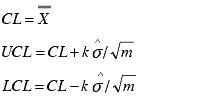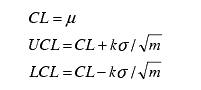Sigma chart control limits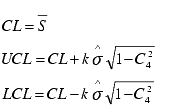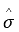is calculated using subgroup standard deviations instead of subgroup ranges. For more information, see Estimated Sigma.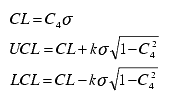NOTE: If subgroup size is variable, m is replaced with mi for ith subgroup limits. When subgroup size is variable, the control limit values will not be the same; therefore, the chart may display `city skyline' type control lines.

## Data Plot Items

• Subgroup Mean (upper chart)

• Subgroup Sigma (lower chart)

• Lower Control Limit (LCL) (optional)

• Upper Control Limit (UCL) (optional)

• Warning lines (optional)

## Descriptive Statistics Displayed

• Lower Control Limit (LCL)

• Upper Control Limit (UCL)

• Center Line (CL)